ISSN: 1070-4698

# Vol. 34

Latest Volume
All Volumes
All Issues

PIER Vol. 34, 313-333, 2001. doi:10.2528/PIER01071201

###Applicability of Combined Microwave and Optical Data for Surface Water Quality Retrievals

Y. Zhang, Jouni Pulliainen, S. Koponen, and M. Hallikainen

This paper presents the applicability of combined microwave (ERS-2 SAR) and optical (Landsat TM) data for surface water quality retrievals. Three surface water quality parameters such as turbidity,Secc hi disk depth (SDD), and suspended sediment concentration (SSC) in the Gulf of Finland are estimated using multivariate approach. The results indicate that the visible bands of TM data are somewhat correlated to SAR signals,b y which SAR can explain 14.4% of the variability in TM2 observations. Multivariate analyses show that SAR has some contribution to derive those parameters if they are somewhat correlated to the water surface oproperties. Although it may be possible to develop algorithms for surface water quality retrievals in which SAR is used as supplementary data to optical observations,it still needs to be refined sufficiently to detect differences within the range of these parameters in the area under study.

PIER Vol. 34, 299-312, 2001. doi:10.2528/PIER01092703

###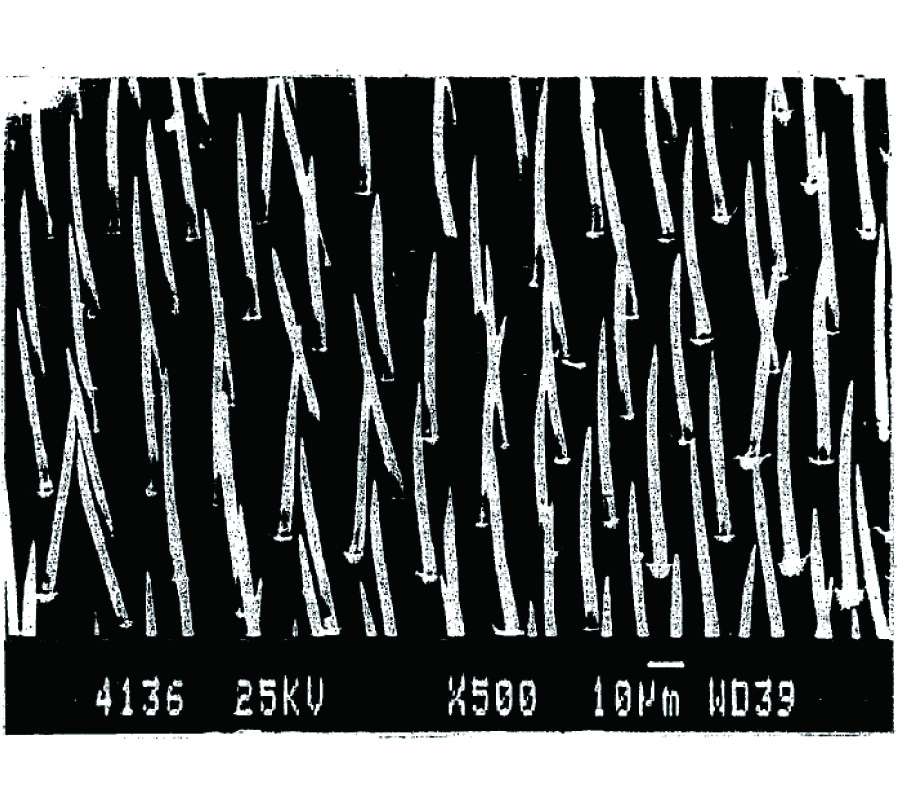Hypothesis of Natural Radar Tracking and Communication Direction Finding Systems Affecting Hornets Flight

Jacob Gavan and Jacob Ishay

The hypothesis of a natural sophisticated RADAR tracking system affecting hornets flight was described, analyzed and developed in previous papers [1, 2] considering their (cuticle) skin complex spike elements arrays shown by electronic microscope pictures. The existence of different spike length arrays and their disposition lead, by analogy with antenna, and radio theory and practice, to the hypothesis of transmitting and receiving phased arrays antennae operating at three different wavelengths in the sub-millimetric bands. The natural photo-and piezo electric generation of energy in the hornets reported previously explain how is generated the Radio Frequency (RF) energy required for the operation of the sub-millimeter wavelength natural RADAR system. However, similar to bats, new computation results show that the operation range of hornets RADAR system is limited to less than 100 m. Recently, investigations have shown that also in the hornet two antennae are located hundreds of spikes which may radiate and detect significant radio energy from their internal photo electric sources and piezoelectric effect. Thus, the existence of three separate sources of radiation and detection enable the application of Direction Finding (DF) communication additional hypothesis. The DF concept can contribute to explain how male hornets track the queens and how workers can be guided to theirnest even forop eration distances up to a few kms. It is possible that the results of the proposed investigations will provide tools to improve real tracking and DF systems performances especially in the yet not sufficiently explored submillimeter wavelength ranges and to find applications to this novel hornet property. Soon will be published results and analysis of experiments contributing to prove this fascinating hypothesis.

PIER Vol. 34, 285-298, 2001. doi:10.2528/PIER01092102

###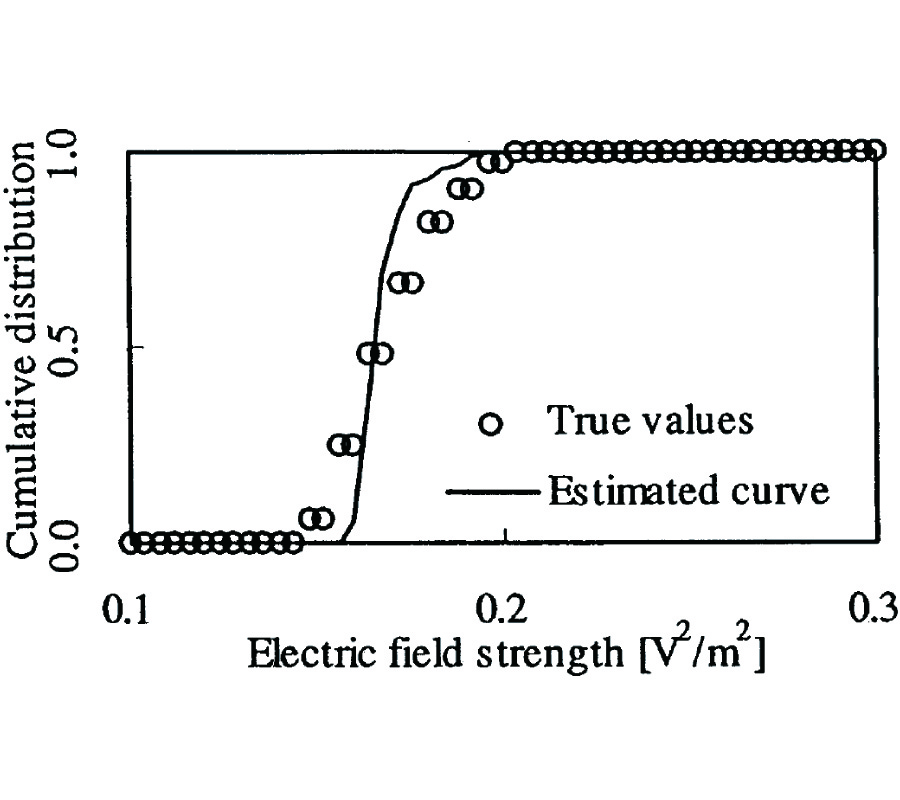A Trial on Hierarchical Extraction of Higher Order Correlation Between Electromagnetic and Sound Waves Around a Vdt Environment --- Practical Use of Background Noise and Probability Prediction

Mitsuo Ohta and H. Ogawa

In this paper, a trial of probabilistic signal processing which is possible to give methodological suggestion to some quantitative measurement method of compound and/or ac-cumulation effect in electromagnetic (abbr. EM) environment is discussed. In order to extract various types of latent interrelation characteristics between many of waved environmental factors (EM and sound waves) leaked from VDT in a real working situation, some extended regression system model reflecting hierarchically not only linear correlation information of the lower order but also nonlinear correlation information of the higher order is firstly introduced. Especially, differing from the previous study, all regression parameters of this model are identified by positively utilizing information of a background EM noise instead of eliminating it. Then, some evaluation method for predicting a whole fluctuation distribution form from sound to EM is newly proposed. Finally, the validity and effectiveness of this proposed method are partly confirmed through some principle experiment too by applying it to the actually observed data leaked by a VDT playing some television games in the room of an actual working environment.

PIER Vol. 34, 271-284, 2001. doi:10.2528/PIER01060603

###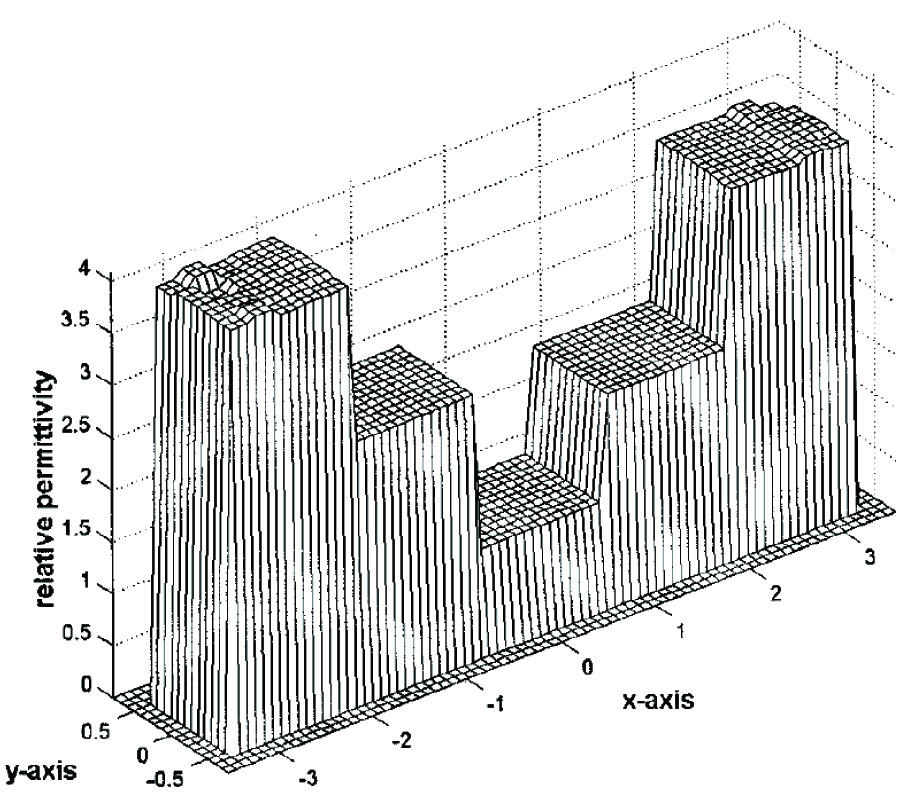Image Reconstruction of Buried Dielectric Cylinders by TE Wave Illumination

C.-C. Chiu and Chun Lin

The inverse scattering of buried dielectric cylinders by transverse electric (TE) wave illumination is investigated. Dielectric cylinders of unknown permittivities are buried in one half space and scatter a group of unrelatedTE waves incident from another half space where the scattered field is recorded. By proper arrangement of the various unrelated incident fields, the difficulties of ill-posedness and nonlinearity are circumvented, and the permittivity distribution can be reconstructedthrough simple matrix operations. The algorithm is basedon the moment methodandthe unrelatedillumination method. Numerical results are given to demonstrate the capability of the inverse algorithm. Goodreconstruction is obtainedev en in the presence of additive random noise in measured data. In addition, the effect of noise on the reconstruction result is also investigated.

PIER Vol. 34, 253-270, 2001. doi:10.2528/PIER01062202

###Analysis of Radiation Characteristics of an Open Circular Waveguide Asymmetrically Covered by a Dielectric Layered Hemi-Spherical Radome

M.-S. Leong, L.-W. Li, X. Ma, P.-S. Kooi, and T. S. Yeo

In this paper, the radiation characteristics of an open circular waveguide asymmetrically covered by a layered dielectric hemi-spherical radome are analyzed. On the waveguide opening, the dominant TE11 wave of the circular waveguide is assumed. The technique of dyadic Green's function is applied to obtain the radiated electromagnetic fields due the circular aperture. Huygens' equivalence principle and the image theory are utilized to simplify the problem. The translational addition theorems of spherical vector wave functions are also employed to make the mathematical representation of the radiated fields compact. Both the exact formulation in the near (radiating-field) zone and the approximate expressions in the far (Fraunhofer) zone of the radiated fields are obtained. Numerical computations are implemented to show the effects of the off-centered source feed asymmetrically covered by the hemi-spherical dielectric radome.

PIER Vol. 34, 219-252, 2001. doi:10.2528/PIER01062201

###EM Fields Inside a Prolate Spheroid Due to a Thin Circular Loop: a Higher-Order Perturbation Approach

L.-W. Li, M. S. Yeo, and M.-S. Leong

This paper presents an alternative analysis of obtaining radiated electromagnetic (EM) fields in a dielectric prolate spheroid using the perturbation technique. A circular loop antenna is used as a radiator on the top of the spheroid. The spheroid is approximated by the first a few terms of the Taylor series expansion (higher-order approximation), and coefficients for transmission and scattered EM fields are found using the perturbation method where the coefficients are also expanded into Taylor series and determined by matching the boundary conditions on the spheroidal dielectric surface. After the approximated coefficients and EM fields are obtained, validity of the approach is discussed and limitations are also addressed.

PIER Vol. 34, 189-218, 2001. doi:10.2528/PIER01061103

###Contrast Source Inversion Method: State of Art

Peter Van den Berg and A. Abubakar

We discuss the problem of the reconstruction of the profile of an inhomogeneous object from scattered field data. Our starting point is the contrast source inversion method, where the unknown contrast sources and the unknown contrast are updated by an iterative minimization of a cost functional. We discuss the possibility of the presence of local minima of the nonlinear cost functional and under which conditions they can exist. Inspired by the successful implementation of the minimization of total variation and other edgepreserving algorithms in image restoration and inverse scattering, we have explored the use of these image-enhancement techniques as an extra regularization. The drawback of adding a regularization term to the cost functional is the presence of an artificial weighting parameter in the cost functional, which can only be determined through considerable numerical experimentation. Therefore, we first discuss the regularization as a multiplicative constraint and show that the weighting parameter is now completely prescribed by the error norm of the data equation and the object equation. Secondly, inspired by the edge-preserving algorithms, we introduce a new type of regularization, based on a weighted L2 total variation norm. The advantage is that the updating parameters in the contrast source inversion method can be determined explicitly, without the usual line minimization. In addition this new regularization shows excellent edge-preserving properties. Numerical experiments illustrate that the present multiplicative regularized inversion scheme is very robust, handling noisy as well as limited data very well, without the necessity of artificial regularization parameters.

PIER Vol. 34, 165-187, 2001. doi:10.2528/PIER01060501

###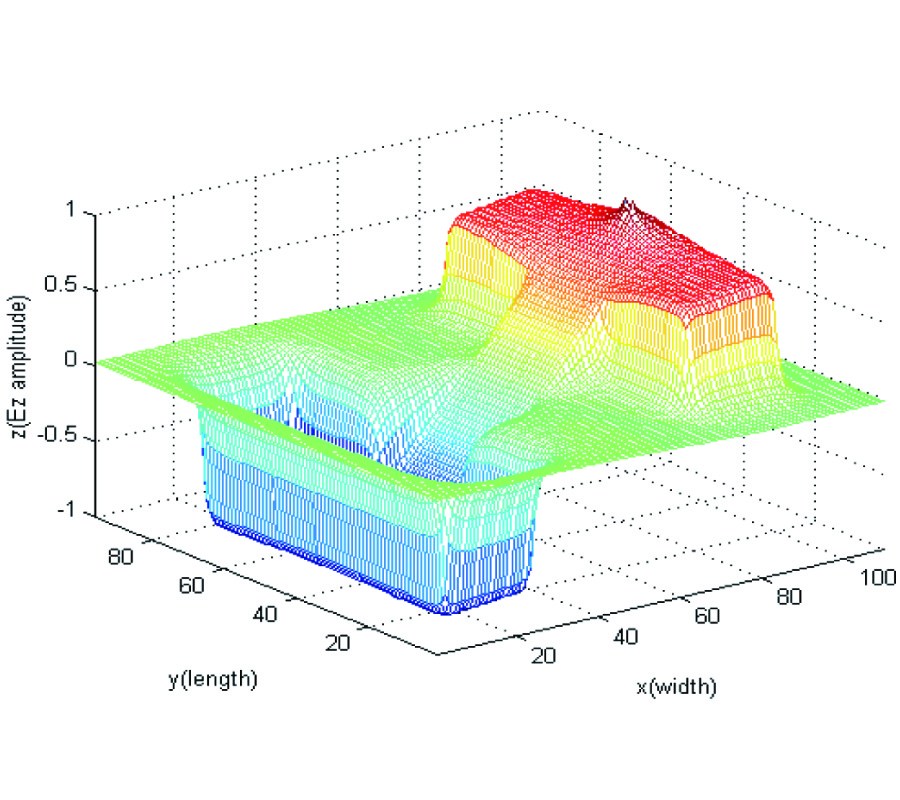Analysis of an h -Shaped Patch Antenna by Using the FDTD Method

S.-C. Gao, L.-W. Li, M.-S. Leong, and Tat Yeo

In this paper, the characteristics of a small antenna using an H-shaped microstrip patch are studied. Significant reduction of antenna size can be realized when the H-shaped patch is used instead of the conventional rectangular microstrip patch antenna. The theoretical analysis is carried out based on the finite-difference timedomain (FDTD) method. The FDTD programs are developed and validated by available measurement results. The effects of various antenna parameters on the resonant frequency and radiation patterns are shown. Several design curves are presented, which are useful for practical antenna design. The electric current distributions on the patch and those on the ground plane are described, together with the results illustrating the electric field distributions under the patch. This antenna is suitable for applications where small size and broad beamwidth are required.

PIER Vol. 34, 143-163, 2001. doi:10.2528/PIER01052406

###Fast and Efficient Analysis of Inset Dielectric Guide Using Fourier Transform Technique with a Modified Perfectly Matched Boundary

H. Jia, Kiyotoshi Yasumoto, and Kuniaki Yoshitomi

A fast and efficient method for analyzing an inset dielectric guide is presented using the Fourier transform technique with a modified perfectly matched boundary. In order to deal with an open region, a novel idea, modified perfectly matched boundary condition (PMB), has been proposed. By introducing the modified PMB, the numerical integral has been avoided and the accuracy of the numerical solution has been improved. Moreover, the singular behavior of the fields at metal edge is taken into account in the analysis. The numerical examples are shown that the convergence of the solution is very fast and the relative error less than 0.07% is attained even if only the first term is considered in the field expansion of the guide. The numerical results of the propagation constants for single- and double-layered inset dielectric guides agree well with those of literatures.

PIER Vol. 34, 117-141, 2001. doi:10.2528/PIER01042402

###Method of Moments Analysis of Electrically Large Thin Square and Rectangular Loop Antennas: Near- and Far-Zone Fields

C.-P. Lim, L.-W. Li, and Mook-Seng Leong

This paper presents a method of moments (MoM) analysis, obtains the non-uniform current distribution in closed form, and computes the resulted radiated patterns in both near and far zones, of square and rectangular loop antennas with electrically larger perimeter. An oblique incident field in its general form is considered in the formulation of the non-uniform current distributions. In the Galerkin's MoM analysis, the Fourier exponential series is considered as the fulldomain basis function series. As a result, the current distributions along the square and rectangular loops are expressed analytically in terms of the azimuth angle for various sizes of large loops. Finally, an alternative vector analysis of the electromagnetic (EM) fields radiated from thin rectangular loop antennas of arbitrary length 2a and width 2b is introduced. This method which employs the dyadic Green's function (DGF) in the derivation of the EM radiated fields makes the analysis general, compact and straightforward in both near- and farzones. The EM radiated fields are expressed in terms of the vector wave eigenfunctions. Not only the exact solution of the EM fields in the near and far zones outside √a2 + b2 are derived by the use of the spherical Bessel and Hankel functions of the first kind respectively, but also the inner regions between a and √a2 + b2 are characterized by both the spherical Bessel and Hankel functions of the first kind. Validity of the numerical results is discussed and clarified.

PIER Vol. 34, 89-115, 2001. doi:10.2528/PIER01032302

###Rigorous Coupled Wave Analysis of Radially and Azimuthally-Inhomogeneous, Elliptical, Cylindrical Systems

John Jarem

Rigorous Coupled Wave Analysis (RCWA) (used for electromagnetic (EM) analysis of planar diffraction gratings) has been applied to solve EM scattering and diffraction problems for spatially inhomogeneous, cylindrical, elliptical systems. The RCWA algorithm and an appropriate method for matching EM boundary conditions in the elliptical system are described herein. Comparisons of the eigenfunctions determined by RCWA (found in spatially homogeneous elliptical regions) and Mathieu functions are presented and shown to agree closely with one another. Numerical results of scattering from a uniform elliptical shell system (excited by an electrical surface current) obtained by using both a Mathieu function expansion method and by using the RCWA algorithm are presented and also shown to agree closely with one another. The RCWA algorithm was used to study EM scattering and diffraction from an elliptical, azimuthally inhomogeneous dielectric permittivity, step profile system. EM field matching and power conservation were shown to hold for this step profile example. A comparison of the EM fields of the step profile elliptical shell example and that of a uniform profile elliptical shell having the same excitation and bulk material parameters (permittivity and permeability) was made and significant differences of the EM fields of the two systems were observed.

PIER Vol. 34, 63-88, 2001. doi:10.2528/PIER01030901

###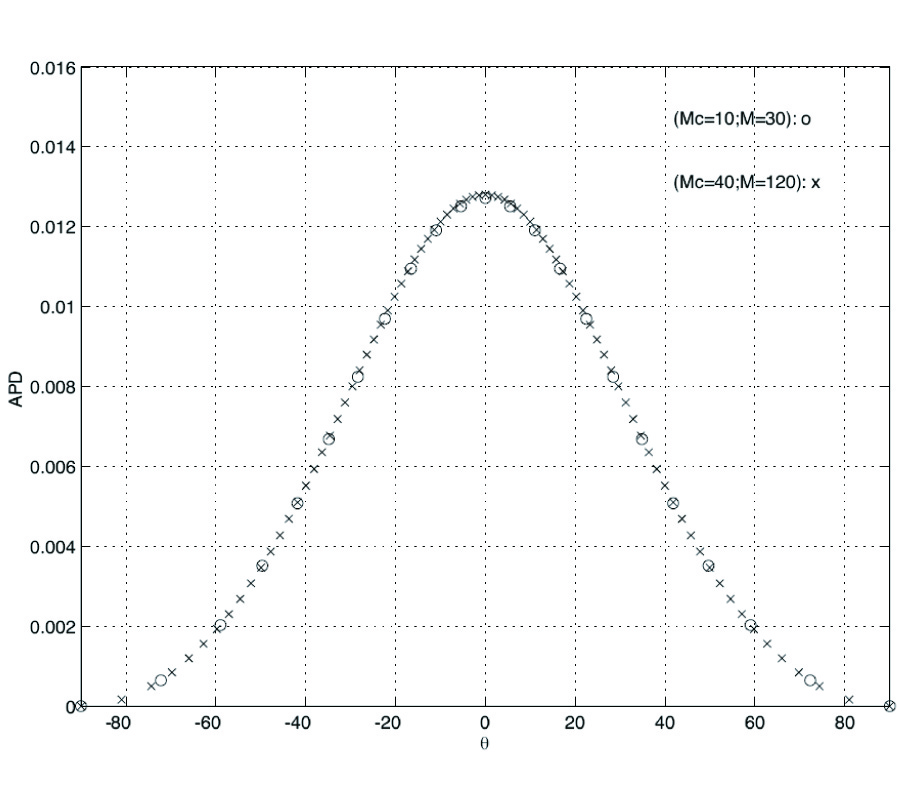Scattering of a Plane Wave by a 1-Dimensional Rough Surface Study in a Nonorthogonal Coordinate System

Richard Dusséaux and Rodrigo De Oliveira

We present a method giving the field scattered by a plane surface with a cylindrical local perturbation illuminated by a plane wave. The theory is based on Maxwell's equations in covariant form written in a nonorthogonal coordinate system fitted to the surface profile. The covariant components of electric and magnetic vectors are solutions of a differential eigenvalue system. A Method of Moments (PPMoM) with Pulses for basis and weighting functions is applied for solving this system in the spectral domain. The scattered field is expanded as a linear combination of eigensolutions satisfying the outgoing wave condition. Their amplitudes are found by solving the boundary conditions. Above a given deformation, the Rayleigh integral is valid and becomes identified with one of covariant components of the scattered field. Applying the PPMo Method to this equality, we obtain the asymptotic field and the scattering pattern. The method is numerically investigated in the far-field zone, by means of convergence tests on the spectral amplitudes and on the power balance criterion. The theory is verified by comparison with results obtained by a rigorous method.

PIER Vol. 34, 29-61, 2001. doi:10.2528/PIER01022605

###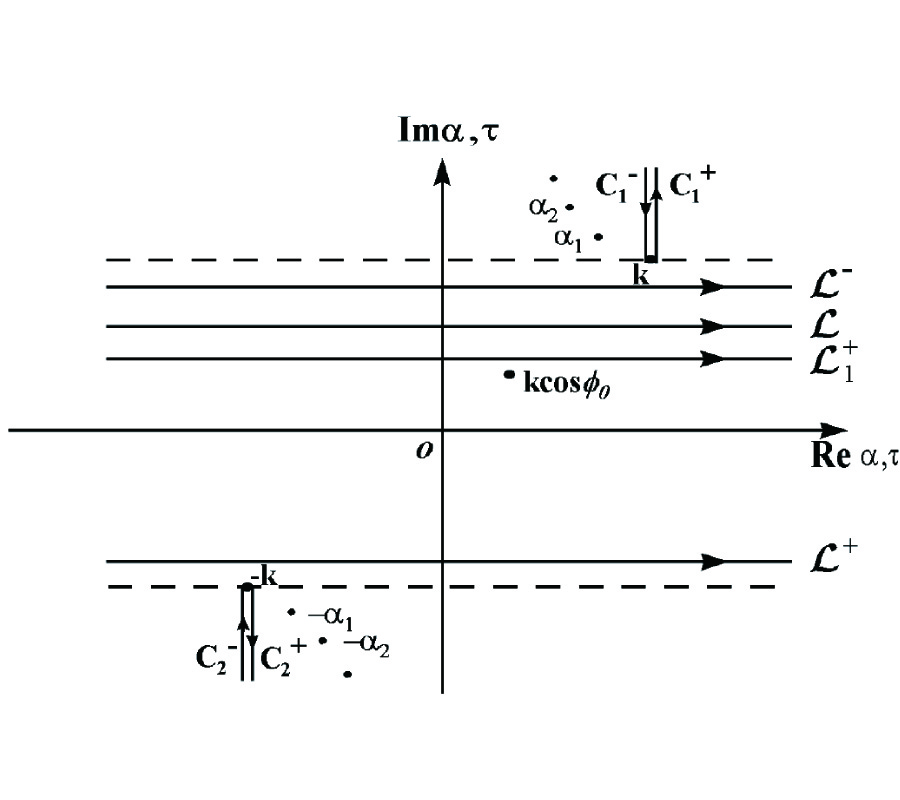Plane Wave Diffraction by Tandem Impedance Slits

B. Polat, Alinur Buyukaksoy, and Gokhan Cinar

The diffraction of E-polarized plane waves by a tandem impedance slit waveguide is investigated rigorously by using the Fourier transform technique in conjunction with the Mode Matching method. This mixed method of formulation gives rise to a scalar Wiener-Hopf equation of the second kind,the solution of which contains infinitely many constants satisfying an infinite system of linear algebraic equations. A numerical solution of this system is obtained for various values of the surface impedances,slit width and the distance between the slits,through which the effect of these parameters on the diffraction phenomenon are studied.

PIER Vol. 34, 1-27, 2001. doi:10.2528/PIER01010501

###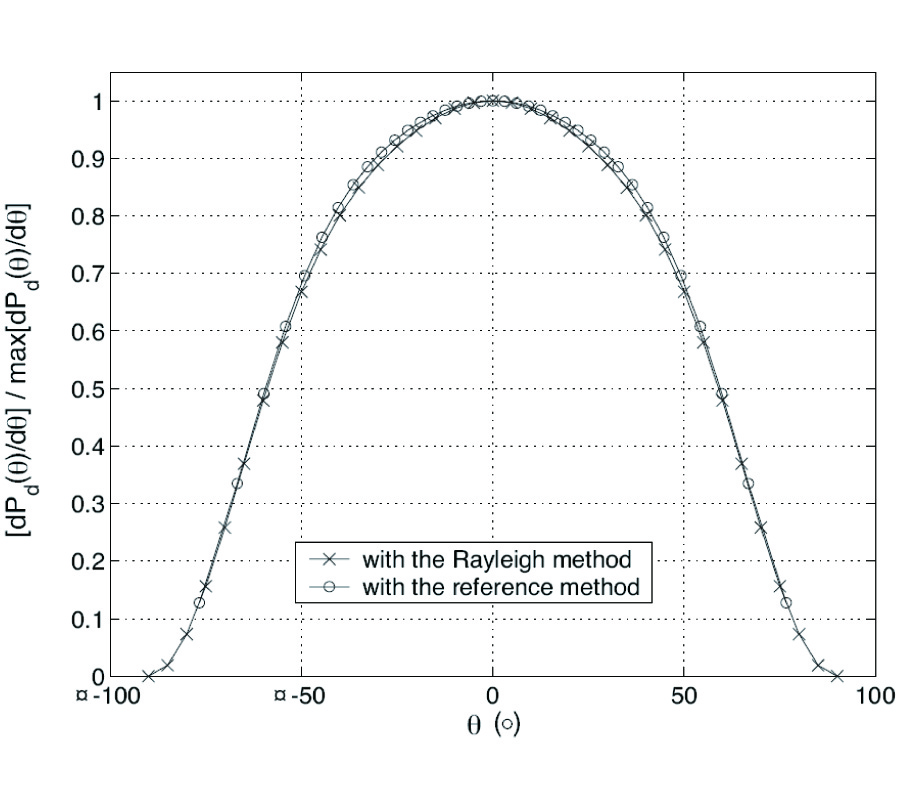Scattering of an E||-Polarized Plane Wave by One-Dimensional Rough Surfaces: Numerical Applicability Domain of a Rayleigh Method in the Far-Field Zone

C. Baudier and Richard Dusséaux

The field scattered by a perfectly conducting plane surface with a perturbation illuminated by an E//-polarized plane wave is determined by means of a Rayleigh method. This cylindrical surface is described by a local function. The scattered field is supposed to be represented everywhere in space by a superposition of a continuous spectrum of outgoing plane waves. A "triangle/Dirac" method of moments applied to the Dirichlet boundary condition in the spectral domain allows the wave amplitudes to be obtained. For a half cosine arch,the proposed Rayleigh method is numerically investigated in the far-field zone,b y means of convergence tests on the spectral amplitudes and on the power balance criterion. We show that the Rayleigh integral can be used for perturbations,the amplitudes of which are close to half the wavelength.